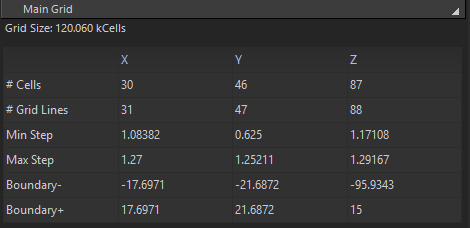# Solved How to get the bounding box of the computational domain of a given simulation from Python?

Let's say I have a simulation object and that its grid is already up-to-date. How can I find the locations of the 6 boundary planes? (or other grid properties, as those shown in the Options window).So I only know how to get the grid settings when the simulation already has results. Don't know if this is what you are looking for, but I'll post it anyway``````import s4l_v1.document as doc
import numpy as np

#get all the simulations
sims = list(doc.AllSimulations)

#select a simulation here
firstSim = sims

#get the results
result_firstSim = firstSim.Results()
result_firstSim = result_firstSim['Overall Field']

#get the grid settings
GridData = result_firstSim
GridData.Update()
GridData = GridData.Data.Grid

#get the axis
X = GridData.XAxis
Y = GridData.YAxis
Z = GridData.ZAxis

#create the matrix that is shown in the options mene
numGridLines = GridData.Dimensions
numCells     = np.array(numGridLines) -1
minStep = np.array([np.min(np.diff(X)),np.min(np.diff(Y)),np.min(np.diff(Z))])*1000
maxStep = np.array([np.max(np.diff(X)),np.max(np.diff(Y)),np.max(np.diff(Z))])*1000
boundaryMin = np.array([X,Y,Z])*1000
boundaryPlus = np.array([X[-1],Y[-1],Z[-1]])*1000

MainGridOptionsArray = np.array([numCells,numGridLines,minStep,maxStep,boundaryMin,boundaryPlus])
``````

Maybe it is also possible to get this data without running the simulation.

I was indeed looking for a way to access this data before running the simulation (so that I can detect in advance that the simulation is likely to be very slow, or to fail, for this or that reason). So your solution does not yet solve my problem.

But thanks a lot for contributing: this piece of code will for sure end up being useful to someone!

one thing you could try is

``````import XCoreModeling as xcm

# get the simulation
sim = doc.AllSimulations

# get bounding box of everything in your simulation
bounding_planes = xcm.GetBoundingBox(list(sim.AllComponents))

# returns a 2-tuple of Vec3 which give the opposite corners of the bounding box